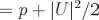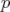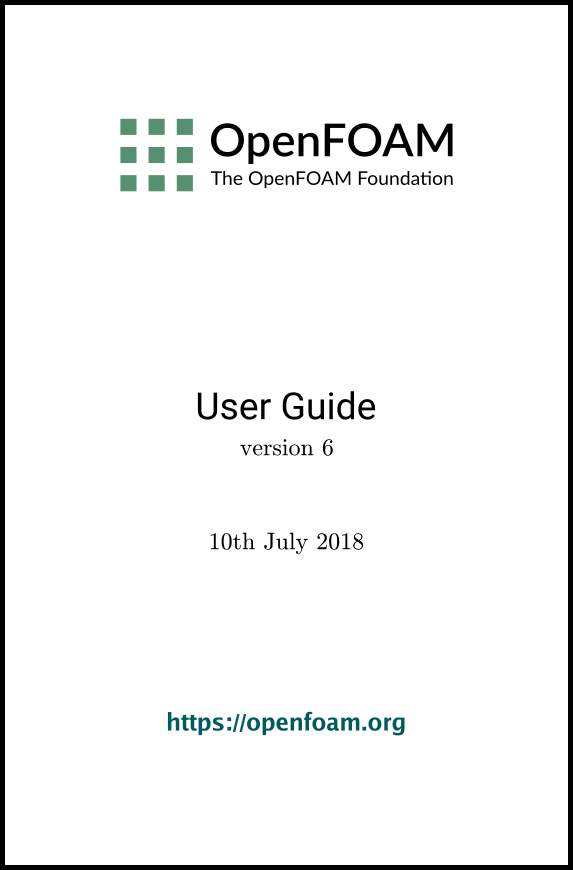## 6.2 Post-processing command line interface (CLI)

In OpenFOAM version 4.0, the ‘non-GUI’ post-processing tools have been unified within a single command line interface (CLI). Post-processing functionality includes data processing, sampling (e.g. probes, graph plotting) visualisation, case control and run-time I/O. Functionality can be executed by:

• conventional post-processing, a data processing activity that occurs after a simulation has run;
• run-time processing, data processing that is performed during the running of a simulation.

Both approaches have advantages. Conventional post-processing allows the user to choose how to analyse data after the results are obtained. Run-time processing offers greater flexibility because it has access to all the data in the database of the run at all times, rather than just the data written during the simulation. It also allows the user to monitor processed data during a simulation and provides a greater level of convenience because the processed results can be available immediately to the user when the simulation ends.

There are 3 methods of post-processing that cover the options described above

• Every solver, e.g. simpleFoam can be configured to include run-time processing.
• The postProcess utility provides conventional post-processing of data written by a simulation.
• Every solver can be run with the -postProcess option, which only executes post-processing, but with additional access to data available on the database for the particular solver.

### 6.2.1 Post-processing functionality

All modes of post-processing access the same functionality implemented in OpenFOAM in the function object framework. Function objects can be listed by running a solver with the -listFunctionObjects option, e.g.

simpleFoam -listFunctionObjects
The list represents the underlying post-processing functionality. Almost all the functionality is packaged into a set of configured tools that are conveniently integrated within the post-processing CLI. Those tools are located in \$FOAM_ETC/caseDicts/postProcessing and are listed by running postProcess with the -list option.

postProcess -list
This produces a list of tools that are described in the following sections.

#### 6.2.1.1 Field calculation

CourantNo
Calculates the Courant Number field from the flux field.
Lambda2
Calculates and writes the second largest eigenvalue of the sum of the square of the symmetrical and anti-symmetrical parts of the velocity gradient tensor.
MachNo
Calculates the Mach Number field from the velocity field.
PecletNo
Calculates the Peclet Number field from the flux field.
Q
Calculates the second invariant of the velocity gradient tensor.
R
Calculates the Reynolds stress tensor field and stores it on the database.
XiReactionRate
Writes the turbulent flame-speed and reaction-rate volScalarFields for the Xi-based combustion models.
components
Writes the component scalar fields (e.g. Ux, Uy, Uz) of a field (e.g. U).
ddt
Calculates the Eulerian time derivative of a field.
div
Calculates the divergence of a field.
enstrophy
Calculates the enstrophy of the velocity field.
flowType
Calculates and writes the flowType of velocity field where: -1 = rotational flow; 0 = simple shear flow; +1 = planar extensional flow.
Calculates the gradient of a field.
mag
Calculates the magnitude of a field.
magSqr
Calculates the magnitude-squared of a field.
randomise
Adds a random component to a field, with a specified perturbation magnitude.
scale
Multiplies a field by a scale factor
streamFunction
Writes the steam-function pointScalarField, calculated from the specified flux surfaceScalarField.
subtract
From the first field, subtracts the remaining fields in the list.
turbulenceFields
Calculates specified turbulence fields and stores it on the database.
turbulenceIntensity
Calculates and writes the turbulence intensity field I.
vorticity
Calculates the vorticity field, i.e. the curl of the velocity field.
wallHeatFlux
Calculates the heat flux at wall patches, outputting the data as a volVectorField.
wallHeatTransferCoeff
Calculates the estimated incompressible flow heat transfer coefficient at wall patches, outputting the data as a volScalarField.
wallShearStress
Calculates the shear stress at wall patches, outputting the data as a volVectorField.
writeCellCentres
Writes the cell-centres volVectorField and the three component fields as volScalarFields; useful for post-processing thresholding.
writeCellVolumes
Writes the cell-volumes volScalarField
writeObjects
Writes out specified objects, e.g. fields, stored on the case database.
yPlus
Calculates the turbulence y+, outputting the data as a yPlus field.

#### 6.2.1.2 Flow rate calculation

flowRateFaceZone
Calculates the flow rate through a specified face zone by summing the flux on patch faces. For solvers where the flux is volumetric, the flow rate is volumetric; where flux is mass flux, the flow rate is mass flow rate.
flowRatePatch
Calculates the flow rate through a specified patch by summing the flux on patch faces. For solvers where the flux is volumetric, the flow rate is volumetric; where flux is mass flux, the flow rate is mass flow rate.
volFlowRateSurface
Calculates volumetric flow rate through a specified triangulated surface by interpolating velocity onto the triangles and integrating over the surface area. Triangles need to be small (�= cell size) for an accurate result.

#### 6.2.1.3 Forces and force coefficients

forceCoeffsCompressible
Calculates lift, drag and moment coefficients by summing forces on specified patches for a case where the solver is compressible (pressure is in units M/(LTˆ2), e.g. Pa).
forceCoeffsIncompressible
Calculates lift, drag and moment coefficients by summing forces on specified patches for a case where the solver is incompressible (pressure is kinematic, e.g. mˆ2/sˆ2).
forcesCompressible
Calculates pressure and viscous forces over specified patches for a case where the solver is compressible (pressure is in units M/(LTˆ2), e.g. Pa).
forcesIncompressible
Calculates pressure and viscous forces over specified patches for a case where the solver is incompressible (pressure is kinematic, e.g. mˆ2/sˆ2).

#### 6.2.1.4 Sampling for graph plotting

singleGraph
Writes graph data for specified fields along a line, specified by start and end points.

#### 6.2.1.5 Lagrangian data

dsmcFields
Calculate intensive fields UMean, translationalT, internalT, overallT from averaged extensive fields from a DSMC calculation.

#### 6.2.1.6 Monitoring minima and maxima

cellMax
Writes out the maximum cell value for one or more fields.
cellMin
Writes out the minimum cell value for one or more fields.
faceMax
Writes out the maximum face value for one or more fields.
faceMin
Writes out the minimum face value for one or more fields.
minMaxComponents
Writes out the minimum and maximum values, by component for non-scalar fields, and the locations where they occur.
minMaxMagnitude
Writes out the minimum and maximum values, by magnitude for non-scalar fields, and the locations where they occur.

#### 6.2.1.7 Numerical data

residuals
For specified fields, writes out the initial residuals for the first solution of each time step; for non-scalar fields (e.g. vectors), writes the largest of the residuals for each component (e.g. x, y, z).
time
Writes run time, CPU time and clock time and optionally the CPU and clock times per time step.

#### 6.2.1.8 Pressure tools

pressureDifferencePatch
Calculates the difference between the average pressures on 2 specified patches.
pressureDifferenceSurface
Interpolates pressures onto 2 specified triangulated surfaces and calculates the difference between the average pressures.
staticPressure
Calculates the pressure field in units M/(LTˆ2) (e.g. Pa) from kinematic pressure by scaling by a specified density.
totalPressureCompressible
Calculates the total pressure field for a case where the solver is compressible (pressure is in units M/(LTˆ2), e.g. Pa).
totalPressureIncompressible
Calculates the total pressure field for a case where the solver is incompressible (pressure is kinematic, e.g. mˆ2/sˆ2).

#### 6.2.1.9 Probes

boundaryCloud
Writes out values of fields at a cloud of points, interpolated to specified boundary patches.
interfaceHeight
Reports the height of the interface above a set of locations. For each location, it writes the vertical distance of the interface above both the location and the lowest boundary. It also writes the point on the interface from which these heights are computed.
internalCloud
Writes out values of fields interpolated to a specified cloud of points.
probes
Writes out values of fields from cells nearest to specified locations.

#### 6.2.1.10 ‘Pluggable’ solvers

icoUncoupledKinematicCloud
Tracks a cloud of parcels driven by the flow of the continuous phase.
scalarTransport
Solves a transport equation for a scalar field.

#### 6.2.1.11 Visualisation tools

streamlines
Writes out files of streamlines with interpolated field data in VTK format.
surfaces
Writes out surface files with interpolated field data in VTK format, e.g. cutting planes, iso-surfaces and patch boundary surfaces.

### 6.2.2 Run-time data processing

When a user wishes to process data during a simulation, they need to configure the case accordingly. The configuration process is as follows, using an example of monitoring flow rate at an outlet patch named outlet.

Firstly, the user should include the flowRatePatch function in functions sub-dictionary in the case controlDict file, using the #includeFunc directive.

functions
{
#includeFunc  flowRatePatch
... other function objects here ...
}
That will include the functionality in the flowRatePatch configuration file, located in the directory hierarchy beginning with \$FOAM_ETC/caseDicts/postProcessing.

The configuration of flowRatePatch requires the name of the patch to be supplied. Option 1 for doing this is that the user copies the flowRatePatch file into their case system directory. The foamGet script copies the file conveniently, e.g.

foamGet flowRatePatch
The patch name can be edited in the copied file to be outlet. When the solver is run, it will pick up an included function in the local case system directory, in precedence over \$FOAM_ETC/caseDicts/postProcessing. The flow rate through the patch will be calculated and written out into a file within a directory named postProcessing.

Option 2 for specifying the patch name is to provide the name as an argument to the flowRatePatch in the #includeFunc directive, using the syntax keyword=entry.

functions
{
#includeFunc  flowRatePatch(name=outlet)
... other function objects here ...
}

In the case where the keyword is field or fields, only the entry is needed when specifying an argument to a function. For example, if the user wanted to calculate and write out the magnitude of velocity into time directories during a simulation they could simply add the following to the functions sub-dictionary in controlDict.

functions
{
#includeFunc  mag(U)
... other function objects here ...
}
This works because the function’s argument U is represented by the keyword field, see \$FOAM_ETC/caseDicts/postProcessing/fields/mag.

Some functions require the setting of many parameters, e.g. forces, forceCoeffs, surfaces, etc. For those functions, it is more reliable and convenient to copy and configure the function using option 1 (above) rather than through arguments.

### 6.2.3 The postProcess utility

The user can execute post-processing functions after the simulation is complete using the postProcess utility. Let us illustrate the use of postProcess using the pitzDaily case from the tutorials directory. The case can be copied, e.g. into the user’s run directory; the user can then go into the case directory, generate the mesh with blockMesh and then run simpleFoam

run
cp -r \$FOAM_TUTORIALS/incompressible/simpleFoam/pitzDaily .
cd pitzDaily
blockMesh
simpleFoam
Now the user can run execute post-processing functions with postProcess. The -help option provides a summary of its use.

postProcess -help
Simple functions like mag can be executed using the -func option; text on the command line generally needs to be quoted ("") if it contains punctuation characters.

postProcess -func "mag(U)"
This operation calculates and writes the field of magnitude of velocity into a file named mag(U) in each time directory. Similarly, the flowRatePatch example can be executed using postProcess.

postProcess -func "flowRatePatch(name=outlet)"
Let us say the user now wants to calculate total pressurefor incompressible flow with kinematic pressure,. The function is available, named totalPressureIncompressible, which the user could attempt first to run as follows.

postProcess -func totalPressureIncompressible
This returns the following error message.

--> FOAM Warning : functionObject pressure: Cannot find required field p
The error message is telling the user that the pressure field p is not loaded; the same is true of the velocity field U. For the function to work, both fields can be loaded as comma separated arguments.

postProcess -func "totalPressureIncompressible(p,U)"
Alternatively the user can load a space separated list of fields using the -fields option, which the function can access.

postProcess -fields "(p U)" -func totalPressureIncompressible
Both options work effectively because the pressure and velocity data is available directly from the files, p and U.

### 6.2.4 Solver post-processing

A more complex example is calculating wall shear stress using the wallShearStress function.

postProcess -fields "(p U)" -func wallShearStress

--> FOAM FATAL ERROR:
Unable to find turbulence model in the database
The message is telling us that the postProcess utility has not constructed the necessary models that the solver, simpleFoam, used when running the simulation, i.e. a turbulence model. This is a situation where we need to post-process (as opposed to run-time process) using the solver with the -postProcess option so that the modelling will be available that the post-processing function needs. Help for this operation can be printed with the following command.

simpleFoam -postProcess -help
It can be seen that the options for a solver with -postProcess are the same as when running postProcess utility. This means that the -func option can be used to execute the wallShearStress function effectively.

simpleFoam -postProcess -func wallShearStress
Note that no fields need to be supplied, either by function arguments ”(p,U)” or using ”-fields (p U)”, because simpleFoam itself constructs and stores the required fields. Functions can also be selected by the #includeFunc directive in functions in the controlDict file, instead of the -func option.
OpenFOAM v6 User Guide - 6.2 Post-processing command line interface (CLI)